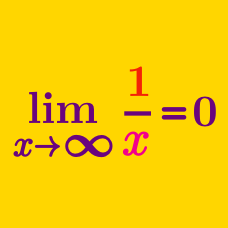Calculus

# Limits of Functions: Level 2 Challenges

$\large \displaystyle \lim_{x\to 0^+} x^x =\, ?$

\begin{aligned} 0.5^{0.5} &=& 0.7071\ldots \\ 0.4^{0.4} &=& 0.6931\ldots \\ 0.3^{0.3} &=& 0.6968\ldots \\ 0.2^{0.2} &=& 0.7247\ldots \\ 0.1^{0.1} &=& 0.7943\ldots \\ \end{aligned}

$\lim_{x\to\infty} \dfrac{\sin x}x = \, ?$

$\Large \lim_{x\rightarrow 0} \frac{|2x-1|-|2x+1|}{x}= \ ?$

$f(x) = \large{\begin{cases} (x^2 - 4)/(x-2), & \text{ if } x < 2 \\ 2, & \text{ if } x = 2 \\ x^3-3x^2 + 2x + 4 , & \text{ if } x > 2 \\ \end{cases} }$

Compute $\displaystyle \lim_{x \to 2} f(x)$.

Given that $f(x) = \dfrac1{x-1}$ and $g(x) = \dfrac3{x^2-3x+2}$, find $\displaystyle \lim_{x\to1} \dfrac{f(x)}{g(x)}$.

$\large \lim_{x \to 0} \, \left \lfloor \dfrac{(\sin x) (\tan x)}{x^2} \right \rfloor = \ ?$

×